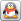收藏本站 | 站点地图 | 繁體中文您现在的位置： 沐阳乐园 >> 文章中心 >> 网络教程 >> 动画实例 >> 正文
 [组图]AS代码制作美丽光影变幻动画 热
AS代码制作美丽光影变幻动画

1.新建一个Flash文档，文件的场景大小设置为590*350.背景为黑色.帧频设置为31，将其另存为blendmode3.fla.

2.打开新建的blendmode3.fla.在主场景中绘制一个38*38大小的园形二维形体，按下shift+F9打开混色器，选择放射状添充，当选择蓝色，如下图所示.3.选中这个园形按下F8键，将其转换为图形符号，命将它的名称命名为Blue.同时注意它的注册点。将注册点置于中心位置。如下图所示。4.确定后我们就创建完成了Blue符号，依照相同的方法，我们需要创建另外4种颜色的小球，在本例中我们创建了如下几种颜色的小球。5．还有一个创建不同颜色小球的简单的方法，选中我们刚创建的蓝色小球，右击选择直接复制。然后在进入到复制后的图形元件内部，进行修改颜色。

6．创建完多个小球之后，我们在主场景保持空的。如果场景上有图形元件要删去。然后按下Ctrl+F8.创建一个新的影片剪辑，我们将它命名为Clip.然后将我们刚刚他建的五个不同颜色的小球元件，从库中拖至clip影片剪辑的帧上，如下图所示。7.ok.完成了这一步之后，返回到主场景中，选中库中我们刚刚创建的clip影片剪辑，右击选择链接。会打开链接标识窗口。如下图所示。8．完成上面的操作之后。选中主场景上的第一帧，按下F9，打开Actionscript面板。我们准备输入代码。

 `//定义中心位置 var cx = 180; var cy = 180; //设定循环20次，准备从库中复制链接id为partical的影片. for (var i = 0; i<20; i++) {     //复制影片剪辑，引用名称为mc.     var mc = this.attachMovie("partical"， "p"+i， i);     with (mc) {         //初始化影片剪辑的位置，注意此时cx，cy是用来调整mc实例的偏移位置的.         _x = cx+Math.random()*60;         _y = cy+Math.random()*60;     }     //针对mc应用融合模式类型"add"     mc.blendMode = "add";     //设定mc实例的角度随机值     mc.tx = random(360);     mc.ty = random(360);     //设定用于mc角度的增量随机值     mc.xtempo = Math.random()/10;     mc.ytempo = Math.random()/10;     //设定mc实例的速度随机值     mc.xd = Math.random()*10+1;     mc.yd = Math.random()*10+1;     mc.x0 = mc._x;     mc.y0 = mc._y;     //跳转到指定的帧，以变换不同颜色的小球上.     mc.gotoAndStop(random(5)+1);     //通过onEnterFrame循环，来让粒子移动.     mc.onEnterFrame = function() {         this.tx += this.xtempo;         this.ty += this.ytempo;         this._x = this.x0+Math.sin(this.tx)*this.xd;         this._y = this.y0+Math.cos(this.ty)*this.yd;     };      }`

9，测试你的影片，你就会看到如下面的效果。`//定义中心位置 var cx = 180; var cy = 180;`

 `//复制影片剪辑，引用名称为mc.     var mc = this.attachMovie("partical"， "p"+i， i);     with (mc) {     //初始化影片剪辑的位置，注意此时cx，cy是用来调整mc实例的偏移位置的.         _x = cx+Math.random()*60;         _y = cy+Math.random()*60;     }`

为粒子实例mc 加入动态属性

 `      //针对mc应用融合模式类型"add"     mc.blendMode = "add";     //设定mc实例的角度随机值     mc.tx = random(360);     mc.ty = random(360);     //设定用于mc角度的增量随机值     mc.xtempo = Math.random()/10;     mc.ytempo = Math.random()/10;     //设定mc实例的速度随机值     mc.xd = Math.random()*10+1;     mc.yd = Math.random()*10+1;     mc.x0 = mc._x;     mc.y0 = mc._y;     //跳转到指定的帧，以变换不同颜色的小球上.     mc.gotoAndStop(random(5)+1);`

 `  //通过onEnterFrame循环，来让粒子移动.     mc.onEnterFrame = function() {         this.tx += this.xtempo;         this.ty += this.ytempo;         this._x = this.x0+Math.sin(this.tx)*this.xd;         this._y = this.y0+Math.cos(this.ty)*this.yd;     };`

 `this._x = this.x0+Math.sin(this.tx)*this.xd; this._y = this.y0+Math.cos(this.ty)*this.yd;`

Ok.在整段代中，我们看到使用融合模式blendmode只有一行，但在这里它显得尤为重要，加上与去掉效果是截然不同的。

 `//定义中心位置 var cx = 0; var cy = 0; //设定循环20次，准备从库中复制链接id为partical的影片. for (var i = 0; i<100; i++) {     //复制影片剪辑，引用名称为mc.     var mc = this.attachMovie("partical"， "p"+i， i);     with (mc) {         //初始化影片剪辑的位置，注意此时cx，cy是用来调整mc实例的偏移位置的.         _x = cx+Math.random()*590;         _y = cy+Math.random()*350;         _xscale = _yscale = 100 * Math.random()*5+1;     }     //针对mc应用融合模式类型"add"     mc.blendMode = "add";     //设定mc实例的角度随机值     mc.tx = random(360);     mc.ty = random(360);     //设定用于mc角度的增量随机值     mc.xtempo = Math.random()/10;     mc.ytempo = Math.random()/10;     //设定mc实例的速度随机值     mc.xd = Math.random()*10+1;     mc.yd = Math.random()*10+1;     mc.x0 = mc._x;     mc.y0 = mc._y;     //跳转到指定的帧，以变换不同颜色的小球上.     mc.gotoAndStop(random(5)+1);     //通过onEnterFrame循环，来让粒子移动.     mc.onEnterFrame = function() {         this.tx += this.xtempo;         this.ty += this.ytempo;         this._x = this.x0+Math.sin(this.tx)*this.xd;         this._y = this.y0+Math.cos(this.ty)*this.yd;     };      }``//定义中心位置 var cx = 0; var cy = 0; //设定循环20次，准备从库中复制链接id为partical的影片. for (var i = 0; i<100; i++) {     //复制影片剪辑，引用名称为mc.     var mc = this.attachMovie("partical"， "p"+i， i);     with (mc) {         //初始化影片剪辑的位置，注意此时cx，cy是用来调整mc实例的偏移位置的.         _x = cx+Math.random()*590;         _y = cy+Math.random()*350;         _xscale = _yscale = 140 * Math.random()*5+1;     }     //针对mc应用融合模式类型"add"     mc.blendMode = "hardlight";     mc.cacheAsBitmap = true;     //设定mc实例的角度随机值     mc.tx = random(360);     mc.ty = random(360);     //设定用于mc角度的增量随机值     mc.xtempo = Math.random()/10;     mc.ytempo = Math.random()/10;     //设定mc实例的速度随机值     mc.xd = Math.random()*10+1;     mc.yd = Math.random()*10+1;     mc.x0 = mc._x;     mc.y0 = mc._y;     //跳转到指定的帧，以变换不同颜色的小球上.     mc.gotoAndStop(random(5)+1);     //通过onEnterFrame循环，来让粒子移动.     mc.onEnterFrame = function() {         this.tx += this.xtempo;         this.ty += this.ytempo;         this._x = this.x0+Math.sin(this.tx)*this.xd;         this._y = this.y0+Math.cos(this.ty)*this.yd;     };      }`

 文章录入：晴天    责任编辑：音乐听听 上一篇文章： 用AS生成盛开的花朵 下一篇文章： 制作流动的曲线
 【字体：小 大】 【发表评论】【加入收藏】【告诉好友】【打印此文】【关闭窗口】
 广告招商网友评论：（只显示最新10条。评论内容只代表网友观点，与本站立场无关！）::发表评论::
 姓 名： 评 分： 1分 2分 3分 4分 5分 评论内容： 严禁发表危害国家安全、政治、黄色淫秽等内容的评论。 用户需对自己在使用本网服务过程中的行为承担法律责任。 本站管理员有权保留或删除评论内容。 评论内容只代表网友个人观点，与本网站立场无关。
 广告招商文章 下载 图片 文字广告| 设为首页 | 加入收藏 | 联系站长 | 友情链接 | 版权申明 | 网站公告 | 管理登陆

 备案/许可证编号: 闽ICP备16020960号-1号　域名创建于2016年8月版权: 沐阳乐园 All Rights Reserved 站长:张萍 邮箱:381415887@qq.com 联系QQ: